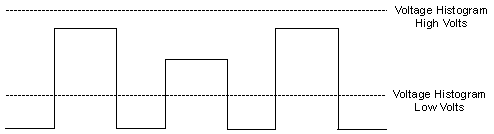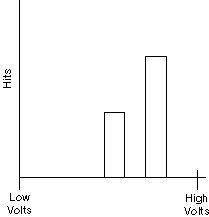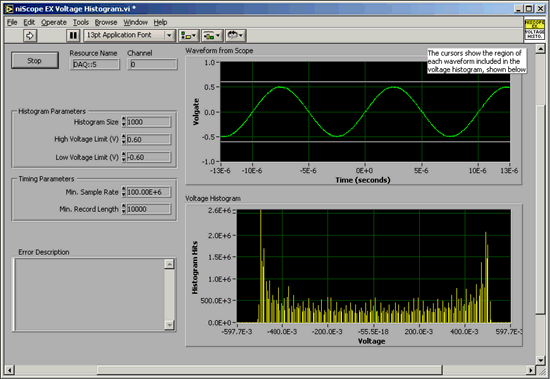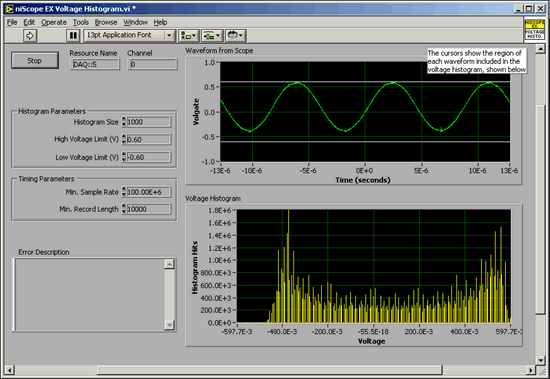# Voltage Histograms

NI High-Speed Digitizers Help (NI-SCOPE)

Edition Date: January 2017

Part Number: 370592AB-01

View Product InfoNI-SCOPE (English | Japanese)

Many scientific and engineering fields require characterization of statistical variations in signal amplitude. Pulse height analysis, a common application in many scientific disciplines, can benefit from voltage histograms. In electronic measurements, signal distortion and quality can also be characterized by voltage histograms. Voltage histograms eliminate the time information from multiple acquisitions and place each point in the acquired waveform into a voltage bin. This is useful for analyzing the statistical amplitude variations of signals. The following figure shows a time-domain waveform sampled by a high-speed digitizer. The second figure shows the corresponding voltage histogram.Note  The higher amplitude pulse occurs twice. The lower amplitude pulse occurs only once.## Voltage Histogram Measurements Application

One application calling for voltage histogram measurements is electronic signal characterization. Signal sources, such as function generators and arbitrary waveform generators, and output amplifiers are characterized in a number of ways. Voltage histograms allow you to promptly characterize the sinusoidal output for voltage offset and distortion, especially zero crossing distortion or crossover distortion.

The voltage histogram of a sine waveform ideally looks like a saddle with the peaks occurring at the maxima and minima of the sine waveform. The saddle is centered at zero if the sine waveform has no DC voltage offset. Any anomalous peaks in the histogram between the maxima and minima reflect nonlinear distortion in the sine waveform. A peak at the center of the voltage histogram reflects zero-crossing distortion, which is a key concern for output amplifier performance.

The two following figures show voltage histograms taken using an NI 5122 high-speed digitizer. The histograms characterize the sine output of two function generators. The sine output in the first figure is relatively distortion-free and the histogram centers at zero. By contrast, the histogram in the second figure is not centered at zero, indicating distortion and voltage drift in the sine output. Thus, while neither histogram shows zero-crossing distortion to be a primary problem, the noisy histogram in the second figure reveals considerable spectral purity degradation in the second function generator.#### Voltage Histogram of 100 kHz 1 Vpk-pk Sine Output from Function Generator 1